occasional meanderings in physics' brave new world

## Monday, April 30, 2007

### Sparring Sparling IV

Since comments on blogs float like corks on the sea, I will bookmark here the interesting remarks of Matti Pitkanen and Carl Brannen on the Abell cluster A586, which is a particularly spherical galaxy cluster. The authors of this recent paper find that, for A586, the ratio of kinetic energy density to potential energy density is $-0.76 \pm 0.05$. This is quite different to the value of $-0.5$ expected from the usual virial theorem.

Ignoring interpretations involving the Dark Force, Carl pointed out that the actual value of $-0.75$ was precisely three times the expected value. This may be explained by a higher value for the speed of light, which fits nicely into the preon particle physics, but the actual value of $c$ is not really relevant.

Kinetic energy goes as $v^2 = v_{1}^{2} + v_{2}^{2} + v_{3}^{2}$ for a 3-vector $v$. Thus for the three speeds of light, forming a 3-vector $(c_1, c_2, c_3)$, the kinetic energies will add. But if we ignore the three speeds, and substitute instead a value which is the length of $(c_1, c_1, c_1)$, then we would have $c = \sqrt{3} c_1$. In the first instance the kinetic energy goes as $\frac{v^2}{c_{1}^{2}}$, while in the second it goes as $\frac{1}{3} \frac{v^2}{c_{1}^{2}}$. This is why kinetic energy at small cosmological scales is incorrectly defined by a factor of 3. Of course, at small scales this doesn't matter.

This links the mass generators of M theory, which obey the tripled Pauli statistics, to the three time coordinates.

### Mass Gap Revisited

There appears to be a lot of work involved in completely solving the mass gap Millennium Problem. The official problem statement, by Jaffe and Witten, asks:

"Prove that for any compact simple gauge group $G$, a non-trivial quantum Yang-Mills theory exists on $\mathbb{R}^4$ and has a mass gap $\Delta > 0$. Existence includes establishing axiomatic properties at least as strong as those cited in [Osterwalder + Schrader, Streater + Wightman]."

Unlike the purely mathematical problems, not all terms in this statement are well defined. What is a Yang-Mills theory? The article suggests that a rigorous formulation of QCD would be adequate. The remaining difficulty with the statement is the one thing that people seem so comfortable with: the use of $\mathbb{R}^4$.

In M theory, as in Algebraic Geometry, we don't like fixing number fields unnecessarily. In fact, since the numbers depend on the class of experimental question, we had better go a long way with the rationals before we even contemplate any kind of continuum limit. Fortunately, Grothendieck understood this a long time ago. Now when we discuss twistor geometry and the division algebras, we understand that these things are simply convenient models for the underlying operadic axioms.

It is still necessary, however, to display in detail how this geometry arises from the axioms. This is why we need Motivic Cohomology, because without it we will never get close to anything looking like a path integral.

## Sunday, April 29, 2007

### Sparring Sparling III

Nigel Cook made an interesting comment about the three times. In particular, he pointed out that "physically, every body which has gained gravitational potential energy, has undergone contraction and time dilation, just as an accelerating body does. This is the equivalence principle of general relativity."

Note that by considering stationary objects in the Sparling metric, in the approximation of one $c$ value, we obtain

$s^2 = c^2 (t^2 + u^2 + v^2)$

which is basically Louise's equation, provided we set a time parameter $T$ to be the length of the time 3-vector. Unfortunately, Lunsford does not appear to have a blog, but here is a link to Nigel's discussion on this matter.

## Saturday, April 28, 2007

### Sparring Sparling II

G. Sparling may like to discuss three time dimensions, but as Louise Riofrio and Alain Connes often like to point out, we should understand how time is emergent, how the arrow of time arises from the expansion of space.

In lessons on special relativity we always use 1-dimensional spaces, because the relative motion of two frames happens along a line, conveniently called an $x$ axis. The Lorentz transformation relates $x$ and $t$ whilst leaving $y$ and $z$ unchanged. The metric considered by Sparling is simply a threefold version of the pair $(x,t)$, namely

$s^2 = x^2 + y^2 + z^2 - t^2 - u^2 - v^2$

where $t$, $u$ and $v$ are all time coordinates. Using imaginary time, which is only proper, the 1-dimensional case looks like the equation for a circle in the complex plane. Similarly, one might interpret the full metric as a 5-sphere in $\mathbb{C}^3$.

But what happened to the speed of light? The metric should really look like

$s^2 = x^2 + y^2 + z^2 - c_{1}^2 t^2 - c_{2}^2 u^2 - c_{3}^2 v^2$

where the $c_i$ form a 3-vector, analogous to the 3-vector for mass in M theory. Deviations from $c_i = 1$ will introduce some ellipticity into the geometry of the hypersurface. Now the homework exercise is to write out $E = m c^2$ three times.

## Friday, April 27, 2007

### Sparring Sparling

Thanks to a commenter at Not Even Wrong we have this link to an article about the work of G. Sparling of twistor fame. The article is about 3 space and 3 time dimensions, and it contains this diagram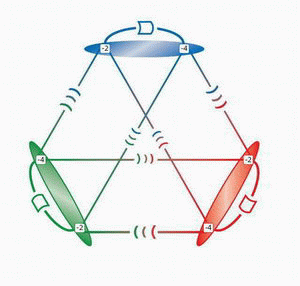which refers to three copies of twistor space. Here is the arxiv link to the paper. The article also mentions triality, Jordan algebras, category theory and condensed matter physics. It feels like Christmas!

### The Maypole

The strange people who have been hanging around this blog for a while will recall a paper by Mulase and Waldron on matrix models and quaternionic graphs.

In particular, T duality appears between the symplectic and orthogonal integrals. This involves a doubling in the size of the matrices being considered. For this reason, it might be interesting to investigate the doubling of matrix sizes in the honeycomb geometry.

Recall that in the 3x3 case, a single central hexagon appears. For 4x4 matrices, there are three central hexagons. In general, the number of hexagons is the sum of $1,2,3, \cdots , N-2$ for $NxN$ matrices, which is equal to $\frac{1}{2} N(N - 1)$. Observe that as $N \rightarrow \infty$ the increase in the number of hexagons obtained by doubling the matrix size is fourfold, since for the $\frac{N}{2}$ case the total is $\frac{1}{8} (N^2 - 2N)$. For any $N$, the number of additional hexagons is given by $\frac{1}{8} (3 N^2 - 2N)$.

By the way, the maypole is a dance (that my childhood ballet class used to perform each year) in which ribbons are knotted.

## Thursday, April 26, 2007

### M Theory Lesson 45

Tao mentions a paper by Speyer on a proof of the honeycomb theorem that uses no representation theory at all. It contains a theorem by Klyachko  which states that the additive problem is solvable for spectra $(\lambda, \mu, \nu)$ iff the multiplicative problem is solvable for $(e^{\lambda}, e^{\mu}, e^{\nu})$.

Kholodenko attacks the Gromov-Witten invariants via the multiplicative problem. The 3x3 relation

$\lambda_1 + \lambda_2 + \lambda_3 + \mu_1 + \mu_2 + \mu_3 = \nu_1 + \nu_2 + \nu_3$

is replaced by the generalised expression

$\lambda_1 + \lambda_2 + \lambda_3 + \mu_1 + \mu_2 + \mu_3 = \nu_1 + \nu_2 + \nu_3 + N (d_1 + d_2 + d_3)$

where the $d_i$ are associated to punctures on a sphere. Let $d$ be the sum of the $d_i$. Fusion rules then belong to quantum cohomology

$\sigma_{a} * \sigma_{b} = \sum_{d,c} q^d C_{ab}^{c} (d) \sigma_{c}$

with a new kind of product for classes. These coefficients give the Gromov-Witten invariants in the genus zero, three point case. In terms of monodromy matrices, Kholodenko writes

$\prod_{i = 1}^{n} \textrm{exp} (2 \pi i \frac{A_i}{d_i}) = \textrm{exp} (2 \pi i I)$

where the $A_i$ are diagonalisable matrices that produce an eigenvalue set.

 A. Klyachko, Lin. Alg. Appl. 319 (2000) 37-59

## Wednesday, April 25, 2007

### Quantum Symmetry

Recall that Coquereaux likes looking at generalised Dynkin diagrams. In a paper on SU(3) graphs there is the example of the $\varepsilon_{21}$ graph, which generalises the diagram for $E_8$. This graph has 24 vertices in three sets of eight.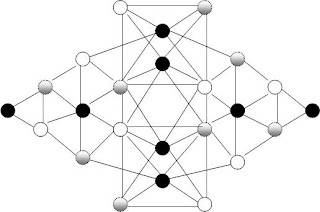Edge orientation is omitted from the diagram. Triality arises from considering the vertex labels mod 3. Complex conjugation corresponds to the symmetry of the central horizontal axis. There is a norm associated to this graph, which takes the value $0.5(1 + \sqrt{2} + \sqrt{6})$. The adjacency matrix for such a graph encodes an associative algebra structure which is compatible with the fusion algebra in a suitable way.

Apparently Ocneanu worked out many details of these quantum symmetries, but his work is largely unpublished. Meanwhile at The Cafe there is an amusing discussion in a comment thread initiated by the probably not-so-innocent David Corfield.

## Tuesday, April 24, 2007

### Internalisation

An arrow f in any category C can be turned into an arrow in some category of categories by taking the functor from the category 2 into C which picks out the arrow f. Similarly, in the category of categories, one can replace a square by a quadruple of squares.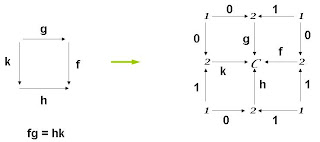The arrow 0 in the diagram represents the source of an arrow, whereas the arrow 1 is the target. Similarly, a triangle is really a hexagon, and so on.

### Triple the Music

The cubist Picasso (1881-1973) painted Les Trois Musiciens in 1921.These days another kind of cubism has influenced music, namely Mazzola's book The Topos of Music (Birkhauser 2002). This mighty tome, first shown to me by Dr Fauser, puts to rest any idea that it is impossible to relate toposes to the real world.

But traditional topos theory only describes classical spaces. Recall that a typical example of a topos is a category of sheaves on some topological space. Since we prefer to study logoses, we might begin simply with the example of Set, a topos with Boolean logic. However, other categories of sheaves are important in twistor theory and it is therefore necessary to generalise all of topos theory in a higher categorical setting.

On the other hand, the sheaf of germs for the celestial 2-sphere is a particular functor on the topological space. The quaternionic analogue uses the 4-sphere and the octonions require the 8-sphere. Only the octonions force us into a consideration of ternary logic, but the quaternions also require non-commutative spaces. So let us begin with the sequence of spheres $S^0$, $S^{2}$, $S^{4}$, $S^{8}$ of dimension $2^n$. A study of generalised sheaves on these spaces should tell us about n-logoses.

## Sunday, April 22, 2007

### M Theory Lesson 44

The construction of honeycombs from overlapping Y pieces may be generalised to include knot crossing pieces. This means working with knotted trivalent graphs for which Kuperberg's spider rules are useful. For example, in the 2x2 case there is the operation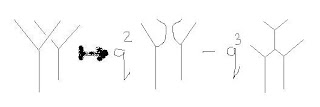and the resolution of one crossing into a >-< diagram appears on the right hand side. There is also a dual relation arising from the opposite crossing.

Thanks to Nigel for this link to a classic paper by J. C. Maxwell involving honeycomb diagrams. In a way, M theory has returned us to this aether, but induced backgrounds in measurement geometry are imposed by the observer and by no means a casual aether in the traditional sense.

## Saturday, April 21, 2007

### Carrying On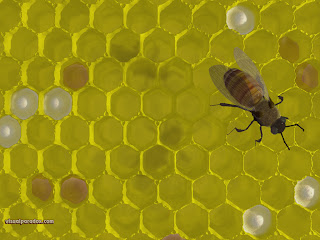A real world honeycomb! Observe that the diagram from lesson 43 is actually a hexagonal tiling of the plane. For larger NxN matrices there are more central hexagons on the largest scale. By tweaking the geometry one could make the pattern regular as in the bee's honeycomb.

## Friday, April 20, 2007

### M Theory Lesson 43

Terence Tao asks the question, what is a quantum honeycomb? Recall that the usual honeycombs for 3x3 complex matrices involve a single hexagon in the plane. Let us resolve the vertices of this hexagon into hexagons.M theory requires octonion Hermitean matrices. Instead of a combination of three matrices resulting in another complex matrix, with 9 external edges, we consider 27 external edges in the triple strand case. Note the scale invariance of the diagram in zooming either inwards or outwards. Altering the local geometry does not affect the rigidity of the pattern. Apologies for the omission of the top Y from the diagram and for the wonky edges.

Drawing a triangle around the triple strand diagram with a vertex at the top of the diagram, we observe that outside the central hexagon there will be 6 pentagons and 3 squares. Thus the cylinder between the triangle and the hexagon happens to have a tiling by the faces of a Stasheff polytope. We could glue two such cylinders together to make a pretty torus geometry.

## Thursday, April 19, 2007

### What's New

Thanks to Marcus at PF for pointing out the abstract of the new paper by Dr S. D. M. White, with the provocative title "Fundamentalist physics: why Dark Energy is bad for Astronomy". Amusingly, despite the title, the author actually thinks Dark Energy is an acceptable theoretical idea.

Meanwhile, I have run down to the library to get hold of Heisenberg's original article Zs. Phys. 33 (1925) 879-893 in English translation (thank you, anonymous) from the volume Sources of Quantum Mechanics, edited by van der Waerden and published by North-Holland in 1967.

As anonymous has noted, Heisenberg was indeed thinking in terms of Fourier transforms, not necessarily commutative, and his original approach to quantum mechanics takes the measurement geometry philosophy seriously. To quote: "instead it seems more reasonable to try to establish a theoretical quantum mechanics, analogous to classical mechanics, but in which only relations between observable quantities occur." Heisenberg's relations look very categorical in nature. His first example is the category of emission frequencies for an electron. In two short pages he argues that the phases are of just as much significance in the quantum case as in the classical. In terms of the real part of expressions $U(n, n - a)exp(i \omega (n, n - a)t)$ he says: "Only the origin of the time scale and hence a phase factor common to all the $U$ is arbitrary and accordingly devoid of physical significance, but the phases of the individual $U$ enter in an essential manner into the [induced scattering moment]."

## Wednesday, April 18, 2007

### M Theory Lesson 42

As Kholodenko points out, Dirac was looking at a multiplication rule for Fourier amplitudes and observed that they did not necessarily commute. In fact, one can derive the quantization relation $[x,p] = i \hbar$ using this observation.

In more recent times Kapranov has studied a non-commutative Fourier transform. He begins by considering cubical paths representing monomials in letters x, y and z.## Tuesday, April 17, 2007

### M Theory Lesson 41

Recall that it is for 3x3 matrices that a Tao hexagon first arises in a diagram representing a sum of three matrices. One segment, or rather one matrix, is composed of the solid lines in the diagramThis contrasts with the 2x2 case, for which only open dagger regions appear, and this may occur in three ways, depending on the orientation of the region.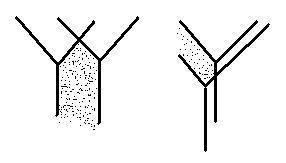It is remarkable to realise that only in the 21st century do we finally return to Dirac's original idea of the q-number. Heisenberg's matrices were always representations of q-numbers, but not true numbers in themselves.

### M Theory Lesson 40

The misuse of common terms may be getting a little out of hand here. We may need to begin inventing words. Let us introduce the term n-logos. This is supposed to be reminiscent of the term n-topos, but more emphasis is being placed on generalised logic.

A 1-logos is like a sheaf, and a 2-logos is more commonly known as a topos (with extra stuff). It is 2-dimensional because it hinges on diagrams made up from squares. As we have found, the structure we need to understand M theory is a 3-logos. This uses parity cubes instead of parity squares, and ternary logic instead of binary logic.

Note that a 2-logos is like a category of 1-logoses, because the canonical example is the topos Set of sets, which are sheaves over a point. But we are defining n-logoses prior to defining categories, which are simply algebras arising from operads. And whereas categories have Euler characteristics, logoses have zeta functions.

### M Theory Lesson 39

On page 29 of the latest version of Carl's never ending paper there is a handy table of left and right handed neutrinos, electrons, up quarks and down quarks. This is eight out of the twelve 3-ribbon diagrams of the Sundance preons.

Carl's table is divided into four pairs, and in each instance the left handed element of the Sundance list may be labelled by the cyclic permutation 231, whereas the right handed element is labelled by 312. These are exactly the permutations of the braid diagrams. The Sundance antiparticles, which are omitted from Carl's table, may also be labelled by the basic cyclic permutations.

The $\sum F_{\chi}$ factor takes a value 0 or 1. The 0 case corresponds to either zero or one twisted strand diagrams, whereas the 1 case corresponds to two or three twisted strands. Recall that these twists are associated with particle charge.

## Monday, April 16, 2007

### M Theory Lesson 38

Carl Brannen (who has a new blog called Mass) likes to talk about 1-circulant matrices, which are of the form

A B C
C A B
B C A

but in M theory we also find 2-circulants

A B C
B C A
C A B

Recall that 1-circulants obey a relation PM = MP for a permutation matrix P, and similarly 2-circulants obey a relation PM = MPP. Lam  studied circulants with rational entries, and in particular circulants M such that M.M is of the form

(d + s) s s
s (d + s) s
s s (d + s)

For 3x3 matrices, the classification of solutions involves cubed roots of unity associated to the cyclotomic polynomial defining the field

$\frac{\mathbb{Q} [x]}{(x^3 - 1)}$

Carl Brannen's 1-circulant matrices often take the form

$A$ $B$ $B^{-1}$
$B^{-1}$ $A$ $B$
$B$ $B^{-1}$ $A$

and we observe that a sum of two such matrices, with B and its inverse interchanged, will result in a matrix of the form studied by Lam et al. Lam's results generalise to all primes p.

 C. W. H. Lam, Lin. Alg. and Appl. 12 (1975) 139-150

## Sunday, April 15, 2007

### Slippery SundayThis is it, folks! Topos theory (a la Isham at least) has made it to the cover of New Scientist. Thanks to The Cafe for the news. Our mathematician friends will doubtless be amused by the prospect of overhearing debates about intuitionism on the bus.

Meanwhile, Christchurch hosts the Armageddon Expo this weekend. People are certainly being entertained. Don't forget to register for GRG18 in July! The conference website now has a preliminary timetable and participant list.

## Saturday, April 14, 2007

### Gravity Probe BIf you are still busy sipping champagne after the MiniBooNE results, grab your hat and get ready for the next installment in the 007 Year of Physics. Gravity Probe B results will be discussed today by C. W. Francis Everitt from Stanford. For the Kiwis, that's late tonight.

As the abstract states, NASA's Gravity Probe B is designed to test two consequences of Einstein's theory, namely (1) the predicted 6.6 arc sec per year geodetic effect due to the motion of the gyroscope through the curved spacetime around the Earth, and (2) the predicted 0.041 arc sec per year frame dragging effect due to the rotating Earth.

Garth at PF has a nice summary of various theories and their predictions.

## Friday, April 13, 2007

### Calabi's Triangle

Calabi found a unique non-equilateral isosceles triangle for which the largest inscribed square can be placed in the triangle in three ways. MathWorld gives us a neat picture: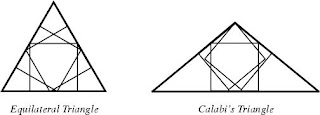I wonder what Calabi was thinking about when he figured this out (see his proof here). MathWorld also tells us about the Biggest Little Hexagon. It is fun to think of uses for these special polygonal geometries.

## Thursday, April 12, 2007

### M Theory Lesson 37

In 2002, Lubos Motl wrote an interesting paper based on Dreyer's work on quasinormal modes and black hole entropy. Carl Brannen has now observed that Lubos makes some intriguing remarks in section 4, after finding that the asymptotics of transmission amplitudes look like

$\tau (\omega) = \frac{1}{e^{\beta \omega} + 3}$

when $j \in 2 \mathbb{Z}$, where $\beta$ is the inverse of the Hawking temperature. Lubos notes that the plus in the denominator is indicative of Fermi statistics, but the strange 3 is something new, which he calls Tripled Pauli Statistics. He says: does it mean that scalar quanta and gravitons near the black hole become (or interact with) J=1 links (triplets) in a spin network that happens to follow Pauli's principle?

Well Lubos, as kneemo has already noted, there might just be something important going on here with our idempotent swapping parity cubes. What do you think?

Tommaso Dorigo and Heather Ray at CV have informative posts about the latest MiniBooNE results, which can be accessed here.The results are consistent with 3 'standard model' massive neutrinos, although the odd low E behaviour remains to be clarified.

Update: Heinrich Paes from Alabama has made the following remark on Not Even Wrong: ...we proposed a spectacular solution to the LSND anomaly involving neutrino shortcuts in extra dimensions, published in Phys.Rev.D72:095017,2005 [hep-ph/0504096]. If you look at Fig. 5 in this paper, you will see that for a choice of the resonant energy in the region 200-300 MeV we not only predicted the small counting rate for electron neutrino events above 475 MeV, but also the large rates in the 300-475 MeV region! While the anomalous effect seen by miniBooNE might have a conventional explanation, it might well be the first hint for extra dimensions of spacetime!

Observe that in our interpretation the extra dimensions would not be 'spacetime', but rather further levels of quantization. It will be interesting to look at this idea further. Here is Matti Pitkanen's post on the matter. Matti points out that there is every reason to expect that MiniBooNE is consistent with LSND, because the latter operated at < 200 MeV which is in the realm of the low E anomalies just observed.

## Wednesday, April 11, 2007

### M Theory Lesson 36

In the paper From Dominoes to Hexagons, D. Thurston proves a nice little theorem. That is, consider a disc with $2n$ endpoints marked on the boundary, such as we see in planar operad diagrams. Then all $n!$ pairings of points marked either in (-) or out (+) are described by a loop free diagram of triple points, which are 6-valent intersections of three strands. Take a look at Thurston's pictures of planar tilings made from diamonds.

Thurston was looking at how planar algebras require more generators than the Temperley-Lieb algebra, which they contain.

### Policies

There has been a spate of Blogging Policy posts today, with one from Louise Riofrio and another from Aaronson. Scott wants to discourage comments from people who have not published papers in peer reviewed journals on the subject in question. Hmmm. Banning people from the arxiv server isn't enough for some people.

The blogging policy here is very simple. Anybody is allowed to comment about the ideas under discussion. We do not discuss irrelevant personal issues such as what so-and-so had for breakfast this morning. Abusive comments will be deleted. Information dissemination is for the benefit of all. This is the Information Age.

Our recommended response to abuse and threats from private sources in public forums is to ignore them. Threats suggesting that we stop discussing new ideas will only encourage louder discussion. If this results in forced silence, may it be noticed.

## Tuesday, April 10, 2007

### Winding Wen

Xiao-Gang Wen at MIT has published some wonderful papers in condensed matter physics. In 2004, along with Levin, he modelled electrons and light with string nets constructed from crossed strings with trivalent vertices and ends which represent the fermions. Numerical simulations show that such string nets can recover Maxwell's equations.### Train Wreck

I hope everybody had a lovely spring/autumn break. Louise Riofrio has been posting about approaching train wrecks. As we all know, Fermilab have scheduled a seminar entitled Initial MiniBooNE Oscillation Results, for April 11 at 11am. That's 3 am Thursday April 12 NZ time. View this event here.

Giving a talk on unification last week was the best thing I ever did: nobody has bothered me all day!

## Monday, April 02, 2007

### Autumn April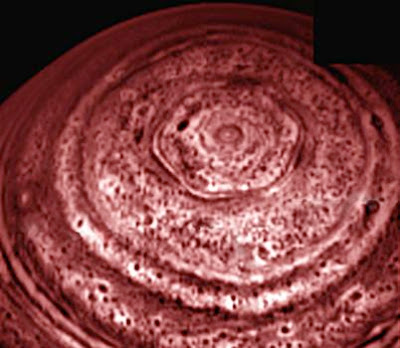I'm no good at April Fool's jokes myself, but I always enjoy having a look at them on the web. Check out NASA's image of the stable hexagonal feature at Saturn's North Pole. Clearly such a thing must be impossible, no?

## Sunday, April 01, 2007

### Coincidence

Recall that Motives are about projective geometry. Naturally then, Motivic Cohomology should be about diagrams with lots of points and lines.We want to build very complicated geometries, perhaps to probe the region close to a Black Hole, from such diagrams. Now we see that dualities abound in motivic pictures. The most basic of these is the one between Space and Time.So there are also singularities in Time, as we observe.

Maybe this is all an April Fool's Joke? Who knows?